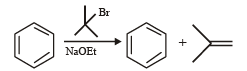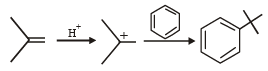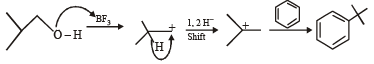Courses

# Test: MCQs (One or More Correct Option): Hydrocarbons | JEE Advanced

## 7 Questions MCQ Test Chemistry 35 Years JEE Main & Advanced Past year Papers | Test: MCQs (One or More Correct Option): Hydrocarbons | JEE Advanced

Description
This mock test of Test: MCQs (One or More Correct Option): Hydrocarbons | JEE Advanced for JEE helps you for every JEE entrance exam. This contains 7 Multiple Choice Questions for JEE Test: MCQs (One or More Correct Option): Hydrocarbons | JEE Advanced (mcq) to study with solutions a complete question bank. The solved questions answers in this Test: MCQs (One or More Correct Option): Hydrocarbons | JEE Advanced quiz give you a good mix of easy questions and tough questions. JEE students definitely take this Test: MCQs (One or More Correct Option): Hydrocarbons | JEE Advanced exercise for a better result in the exam. You can find other Test: MCQs (One or More Correct Option): Hydrocarbons | JEE Advanced extra questions, long questions & short questions for JEE on EduRev as well by searching above.
*Multiple options can be correct
QUESTION: 1

### Which one of the following has the smallest heat of hydrogenation per mole? (1993)

Solution:

TIPS/Formulae : Heat of hydrogenation is related to stability of molecules; higher the stability, lower is the heat of hydrogenation.
Butadiene, CH2 = CHCH = CH2 has two double bonds so its heat of hydrogenation will be more than the other three.
Alkenes follow the following order of stability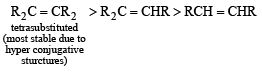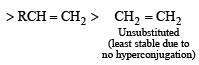Thus here stability order of the given monoalkenes is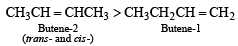NOTE : The trans-2-butene is more stable than the cis-because in the cis-isomer the two bulky groups are crowded together with the result it has more van der Waal’s strain than the trans-isomer.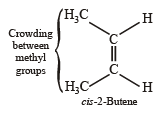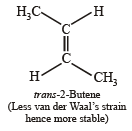*Multiple options can be correct
QUESTION: 2

### Toluene, when treated with Br2/Fe, gives p-bromotoluene as the major product because CH3 group (1999 - 3 Marks)

Solution:

Hyperconjugation in toluene activates the benzene ring for electrophilic substitution.

*Multiple options can be correct
QUESTION: 3

###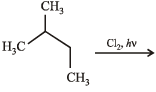N(isomeric products) ; C5H11Cl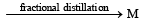(isomeric products) Identify N and M (2006 - 5M,) Identify N and M (2006 - 5M, –1)

Solution: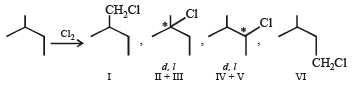So, the value of N will be 1 + 2 + 2 + 1 = 6.
Since enantiomers have nearly same physical properties, II and III as well as IV and V can’t be separated, hence the number of isomers (M) will be 1 + 1 + 1 + 1 = 4.

*Multiple options can be correct
QUESTION: 4

Among P, Q, R and S, the aromatic compound(s) is/are (JEE Advanced 2013-I)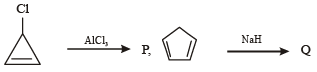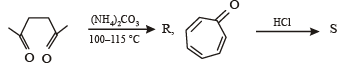Solution: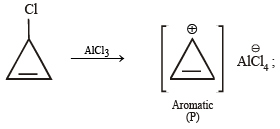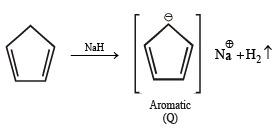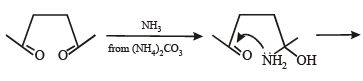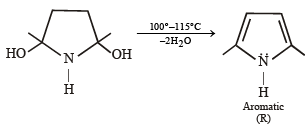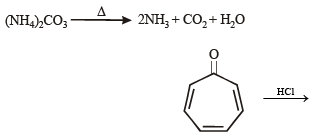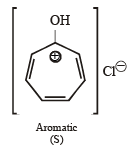Note : P has 2 p electrons, while Q, R & S have 6 p electrons each. Hence all the 4 are aromatic.

*Multiple options can be correct
QUESTION: 5

In the following reaction, the major product is (JEE Adv. 2015)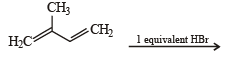Solution: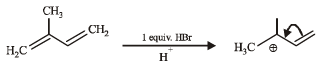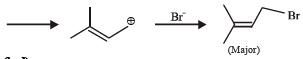*Multiple options can be correct
QUESTION: 6

Compound(s) that on hydrogenation produce(s) optically inactive compound(s) is (are) (JEE Adv. 2015)

Solution: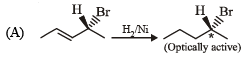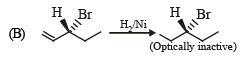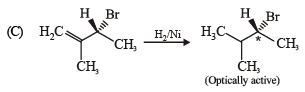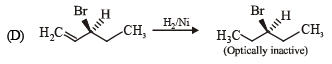*Multiple options can be correct
QUESTION: 7

Among th e followin g, r eaction(s) wh ich gives(give) tert-butyl benzene as the major product is(are) (JEE Adv. 2016)

Solution: